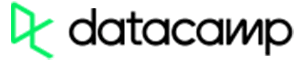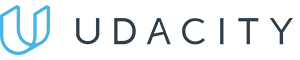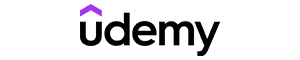Code has been added to clipboard!

# Learn JavaScript Number Format: ParseInt Explained

Published Aug 8, 2017
Updated Oct 15, 2019

While holding numeric values is similar to holding string values, there are some differences. In this tutorial, you will learn about various JavaScript number formats.

You will learn how to convert numbers to strings, how to display exponentials, as well as decimals. You will also understand how to convert variables to numbers, using the most popular methods. We will cover JavaScript parseInt method and explain how to make JavaScript integer as well.

## JavaScript Number Format: Main Tips

• Several JavaScript number format methods are offered with this language that you can use to manipulate numeric values.
• Each number method returns a new value instead of changing the one being used for the method.
• Every JavaScript number format method may be used on any type of number, including literals, variables, expressions.

## Methods Used For Numbers

There are a few JavaScript number format methods to keep in mind if you want to manipulate and change numeric values.

These are the go-to methods while working with numeric values:

Method Description
Number() Return number converted from its argument
parseFloat() Parse its argument and return a float
parseInt() Parse its argument and returns an integer

However, some JavaScript number format methods are specifically used on numbers only. See the table below:

Method Description
toString() Return number as string
toExponential() Return string with number rounded and written with exponential notation.
toFixed() Return string with number rounded and written using a specified number of decimals.
toPrecision() Return string with a number written with a specified length
valueOf() Return number as a number

#### toString()

`toString()` turns the numeric value into a string (a sequence of characters). It can be used with literals, variables or expressions:

Example
``````var a = 653;
a.toString();
(653).toString();
(600 + 53).toString();``````

#### toExponential()

`toExponential()` turns numbers into strings as well, but in addition to that, the number that is returned is also rounded and written with exponential notation.

You also have access to a parameter, which is used to define the number of digits after a decimal point:

Example
``````var a = 4;
a.toExponential();
a.toExponential(2);
a.toExponential(4);
a.toExponential(6);``````

#### toFixed()

The JavaScript `toFixed()` also turns the number into a string, but with a specific amount of decimals this time. The number of decimals should be specified in the parentheses after the method's name:

Example
``````var a = 4.236;
a.toFixed(0);
a.toFixed(1);
a.toFixed(3);
a.toFixed(5);``````

Note: This method is useful for working with payments, where you only need two decimals after the decimal point.

#### toPrecision()

The `toPrecision()` also turns the number into a string, but with a specified length. The length is specified inside the parentheses after the method's name. It is quite similar to JavaScript `toFixed()` method but counts all numbers, not only decimals.

See how it works:

Example
``````var x = 4.236;
x.toPrecision();
x.toPrecision(2);
x.toPrecision(4);
x.toPrecision(6);``````Pros
• Easy to use with a learn-by-doing approach
• Offers quality content
• Gamified in-browser coding experience
• The price matches the quality
• Suitable for learners ranging from beginner to advanced
Main Features
• Free certificates of completion
• Focused on data science skills
• Flexible learning timetablePros
• Simplistic design (no unnecessary information)
• High-quality courses (even the free ones)
• Variety of features
Main Features
• Nanodegree programs
• Suitable for enterprises
• Paid Certificates of completionPros
• Easy to navigate
• No technical issues
• Seems to care about its users
Main Features
• Huge variety of courses
• 30-day refund policy
• Free certificates of completion

## Converting Variables to Numbers

If you find yourself in need to turn a variable into a number, these are the three methods you can use:

• `Number()`
• `parseInt()`
• `parseFloat()`

Note: these are global JavaScript methods, not number methods. Therefore, they can be used everywhere, not only on numeric values.

#### Number()

`Number()` method is widely used to convert JavaScript variables into numbers. Although, if a number cannot be returned, the program will return `NaN` (Not a Number).

Example
``````Number(true)
Number(false)
Number("12")
Number("12.24")
Number("12 24")
Number("12,24")
Number("Tim")``````

Note: if this value is used on a Date() object, this method will return the amount of milliseconds that have passed since the date of 01.01.1970. This date is picked by Unix engineers as a uniform starting date for computers and programs.

#### parseInt()

If you want to create a JavaScript integer, you should use `parseInt()`. It works by parsing a string and then returning the number. Spaces may be present in the string, but only the first number will be returned.

Example
``````parseInt("21");
parseInt("21.32");
parseInt("21 32 42");
parseInt("21 days");
parseInt("day 21");``````

Note: if there is no JavaScript integer that can be converted, NaN (Not a Number) is returned.

#### parseFloat() Method

`parseFloat()` method works by parsing a string and then returning a floating point number. Spaces may be present in the string, but only the first number will be returned.

Example
``````parseFloat("21");
parseFloat("21.14");
parseFloat("21 14 34");
parseFloat("21 day");
parseFloat("day 21");``````

Note: if there is no JavaScript integer that can be converted, NaN (Not a Number) is returned.

#### valueOf()

`valueOf()` is used to return a number as a number. When looking at JavaScript code, numbers may be primitive values (`typeOf = number`) or objects (`typeOf = object`). The method called `valueOf()` is used internally to turn number objects into primitive values.

Example
``````var a = 246;
a.valueOf();
(246).valueOf();
(200 + 46).valueOf();``````

Note: All data types in JavaScript have valueOf() and toString() methods.

## JavaScript Number Format: Summary

• JavaScript number format can be changed through various methods into multiple different values.
• Most popular methods are `Number()`, `parseInt()`, and `parseFloat()`.
• If you want JavaScript to convert to integer, you should use `parseInt()` method.
• Each time JavaScript converts to integer, it returns a new value instead of rewriting the old one.

Days
Hours
Minutes
Seconds